# Would you rather

Someone texted me the following image and asked for my response.So what would I rather do?

• Flip 3 coins and win if all match
• Roll 3 dice and win if none match

I would rather do the one with the highest probability of happening. So let’s calculate that using R.

There are two ways to solve problems like this. One is to use the appropriate probability distribution and calculate it mathematically. The other is to enumerate all possible outcomes and find the proportion of interest. Let’s do the latter first.

We’ll use the expand.grid function to create a data frame of all possible outcomes of flipping 3 coins. All we have to do is give it 3 vectors representing coins. It’s such a small sample space we can eyeball it and see the probability of getting all heads or all tails is 2/8 or 0.25.

s1 <- expand.grid(c("H", "T"), c("H", "T"), c("H", "T"))
s1
##   Var1 Var2 Var3
## 1    H    H    H
## 2    T    H    H
## 3    H    T    H
## 4    T    T    H
## 5    H    H    T
## 6    T    H    T
## 7    H    T    T
## 8    T    T    T

If we wanted to use R to determine the proportion, we could use apply to apply a function to each row and return the number of unique values, and then find the proportion of 1s. (1 means there was only one unique value: all “H” or all “T”)

count1 <- apply(s1, 1, function(x)length(unique(x)))
mean(count1 == 1)
##  0.25

We can do the same with rolling 3 dice. Give expand.grid 3 dice and have it generate all possible results. This will generate 216 possibilities, so there’s no eyeballing this for an answer. As before, we’ll apply a function to each row and determine if there are any duplicates. The anyDuplicated function returns the location of the first duplicate within a vector. If there are no duplicates, the result is a 0, which means we just need to find the proportion of 0s.

s2 <- expand.grid(1:6, 1:6, 1:6)
count2 <- apply(s2, 1, anyDuplicated)
mean(count2 == 0)
##  0.5555556

An easier way to do that is to use permutation calculations. There are $$6^3 = 216$$ possible results from rolling 3 dice. Furthermore there are $$6 \cdot 5 \cdot 4 = 120$$ possible non-matching results. There are 6 possibilities for the first die, only 5 for the second, and 4 for the third. We can then divide to find the probability: $$120/216 = 0.55$$.

Clearly we’d rather roll the dice (assuming fair coins and fair dice).

We can also answer the question using the binomial probability distribution. The binomial distribution is appropriate when you have:

• two outcomes (Heads vs Tales, no match vs One or more match)
• independent events
• same probability for each event

The dbinom function calculates probabilities of binomial outcomes. Below we use it to calculate the probability of 0 heads (3 tails) and 3 heads, and sum the total. The x argument is the sum of “successes”, for example 0 heads (or tails, whatever you call a “success”). The size argument is the number of trials, or coins in this case. The prob argument is the probability of success on each trial, or for each coin in this case.

dbinom(x = 0, size = 3, prob = 0.5) +
dbinom(x = 3, size = 3, prob = 0.5)
##  0.25

We can also frame the dice rolling as a binomial outcome, but it’s a little trickier. Think of rolling the dice one at a time:

• It doesn’t matter what we roll the first time. We don’t care if it’s a 1 or 6 or whatever. We’re certain to get something, so the probability is 1.
• The second role is a “success” if it does not match the first role. That’s one dice roll (size = 1) where success (x = 1) happens with probability of 5/6.
• The third and final roll is a “success” if it doesn’t match either of the first two rolls. That’s one dice roll (size = 1) where success (x = 1) happens with probability of 4/6.

Notice we don’t add these probabilities but rather multiply them.

1 * dbinom(x = 1, size = 1, prob = 5/6) *
dbinom(x = 1, size = 1, prob = 4/6)
##  0.5555556

We added the coin flipping probabilities because they each represented mutually exclusive events. They cannot both occur. There is one way to get 0 successes and one way to get 3 successes. Together they sum to the probability of all matching (ie, all heads or all tales).

We multiplied the dice rolling probabilities because they each represented events that could occur at the same time, and they were conditional if we considered each die in turn. This requires the multiplication rule of probability.

# Fixing the p-value note in a HTML stargazer table

If you insert a stargazer table into R Markdown that outputs an HTML file, you may get a p-value note that looks like this:

p<0.1; p<0.05; p<0.01

What should be displayed is this:

*p<0.1; **p<0.05; ***p<0.01

This is the legend for understanding the “stars” in the regression table. What’s happening is the asterisks are being treated as markdown code, which is adding italics and bolding to the note instead of simply showing the asterisks verbatim. (Recall that in Markdown surrounding text with one asterisk adds italics and surrounding text with two asterisks adds bolding.)

To fix this we can add the following two arguments to the stargazer function:

notes.append = FALSE

notes = c("<sup>&sstarf;</sup>p<0.1; <sup>&sstarf;&sstarf;</sup>p<0.05; <sup>&sstarf;&sstarf;&sstarf;</sup>p<0.01")

&sstarf; is an HTML entity for a star character. notes.append = FALSE says to NOT append the note but rather replace the existing note. The notes argument specifies the note we want to add using the &sstarf; HTML entity.

# Most useful tests for an ANCOVA model

In his book Regression Modeling Strategies, 2nd Ed, Frank Harrell provides a list of what he calls the “most useful tests” for a 2-level factor $$\times$$ numeric model (Table 2.2, p. 19). This is often called an Analysis of Covariance, or ANCOVA. The basic idea is we have a numeric response with substantial variability and we seek to understand the variability by modeling the mean of the response as a function of a categorical variable and a numeric variable.

Let’s simulate some data for such a model and then see how we can use R to carry out these tests.

n <- 400
set.seed(1)
sex <- factor(sample(x = c("f", "m"), size = n, replace = TRUE))
age <- round(runif(n = n, min = 18, max = 65))
y <- 1 + 0.8*age + 0.4*(sex == "m") - 0.7*age*(sex == "m") + rnorm(n, mean = 0, sd = 8)
dat <- data.frame(y, age, sex)


The data contain a numeric response, y, that is a function of age and sex. I set the “true” coefficient values to 1, 0.8, 0.4, and -0.7. They correspond to $$\beta_0$$ through $$\beta_3$$ in the following model:

$y = \beta_0 + \beta_1 age + \beta_2 sex + \beta_3 age \times sex$

In addition the error component is a Normal distribution with a standard deviation of 8.

Now let’s model the data and see how close we get to recovering the true parameter values.

mod <- lm(y ~ age * sex, dat)
summary(mod)

##
## Call:
## lm(formula = y ~ age * sex, data = dat)
##
## Residuals:
##      Min       1Q   Median       3Q      Max
## -23.8986  -5.8552  -0.2503   6.0507  30.6188
##
## Coefficients:
##             Estimate Std. Error t value Pr(>|t|)
## (Intercept)  0.27268    1.93776   0.141    0.888
## age          0.79781    0.04316  18.484   <2e-16 ***
## sexm         2.07143    2.84931   0.727    0.468
## age:sexm    -0.72702    0.06462 -11.251   <2e-16 ***
## ---
## Signif. codes:  0 '***' 0.001 '**' 0.01 '*' 0.05 '.' 0.1 ' ' 1
##
## Residual standard error: 8.661 on 396 degrees of freedom
## Multiple R-squared:  0.7874, Adjusted R-squared:  0.7858
## F-statistic:   489 on 3 and 396 DF,  p-value: < 2.2e-16


While the coefficient estimates for age and the age $$\times$$ sex interaction are pretty close to the true values, the same cannot be said for the intercept and sex coefficients. The residual standard error of 8.661 is close to the true value of 8.

We can see in the summary output of the model that four hypothesis tests, one for each coefficient, are carried out for us. Each are testing if the coefficient is equal to 0. Of those four, only one qualifies as one of the most useful tests: the last one for age:sexm. This tests if the effect of age is independent of sex and vice versa. Stated two other ways, it tests if age and sex are additive, or if the age effect is the same for both sexes. To get a better understanding of what we’re testing, let’s plot the data with fitted age slopes for each sex.

library(ggplot2)
ggplot(dat, aes(x = age, y = y, color = sex)) +
geom_point() +
geom_smooth(method="lm")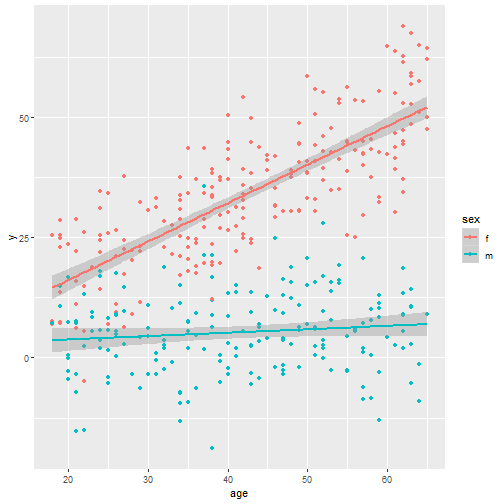Visually it appears the effect of age is not independent of sex. It seems more pronounced for females. Is this effect real or maybe due to chance? The hypothesis test in the summary output for age:sexm evaluates this. Obviously the effect seems very real. We are not likely to see such a difference in slopes this large if there truly was no difference. It does appear the effect of age is different for each sex. The estimate of -0.72 estimates the difference in slopes (or age effect) for the males and females.

The other three hypothesis tests are not very useful.

• Testing if the Intercept is 0 is testing whether y is 0 for females at age 0.
• Testing if age is 0 is testing whether age is associated with y for males.
• Testing if sexm is 0 is testing whether sex is associated with y for subjects at age 0.

Other more useful tests, as Harrell outlines in Table 2.2, are as follows:

• Is age associated with y?
• Is sex associated with y?
• Are either age or sex associated with y?

The last one is answered in the model output. That’s the F-statistic in the last line. It tests whether all coefficients (except the intercept) are equal to 0. The result of this test is conclusive. At least one of the coeffcients is not 0.

To test if age is associated with y, we need to test if both the age and age:sexm coefficents are equal to 0. The car package by John Fox provides a nice function for this purpose called linearHypothesis. It takes at least two arguments. The first is the fitted model object and the second is a vector of hypothesis tests. Below we specify we want to test if “age = 0” and “age:sexm = 0”

library(car)
linearHypothesis(mod, c("age = 0", "age:sexm = 0"))

## Linear hypothesis test
##
## Hypothesis:
## age = 0
## age:sexm = 0
##
## Model 1: restricted model
## Model 2: y ~ age * sex
##
##   Res.Df   RSS Df Sum of Sq      F    Pr(>F)
## 1    398 55494
## 2    396 29704  2     25790 171.91 < 2.2e-16 ***
## ---
## Signif. codes:  0 '***' 0.001 '**' 0.01 '*' 0.05 '.' 0.1 ' ' 1


The result is once again conclusive. The p-value is virtually 0. It does indeed appear that age is associated with y.

Likewise, to test if sex is associated with y, we need to test if both the sex and age:sexm coefficents are equal to 0.

linearHypothesis(mod, c("sexm = 0", "age:sexm = 0"))

## Linear hypothesis test
##
## Hypothesis:
## sexm = 0
## age:sexm = 0
##
## Model 1: restricted model
## Model 2: y ~ age * sex
##
##   Res.Df    RSS Df Sum of Sq     F    Pr(>F)
## 1    398 119354
## 2    396  29704  2     89651 597.6 < 2.2e-16 ***
## ---
## Signif. codes:  0 '***' 0.001 '**' 0.01 '*' 0.05 '.' 0.1 ' ' 1


As expected this test confirms that sex is associated with y, just as we specified when we simulated the data.

Now that we have established that age is associated with y, and that the association differs for each sex, what exactly is that association for each sex? In other words what are the slopes of the lines in our plot above?

We can sort of answer that with the model coefficients.

round(coef(mod),3)

## (Intercept)         age        sexm    age:sexm
##       0.273       0.798       2.071      -0.727


That corresponds to the following model:

$y = 0.273 + 0.799 age + 2.071 sex – 0.727 age \times sex$

When sex is female, the fitted model is

$y = 0.273 + 0.799 age$

This says the slope of the age is about 0.8 when sex is female.

When sex is male, the fitted model is

$y = (0.273 + 2.071) + (0.797 – 0.727) age$
$y = 2.344 + 0.07 age$

This says the slope of the age is about 0.07 when sex is male.

How certain are we about these estimates? That’s what standard error is for. For the age slope estimate for females the standard error is provided in the model output for the age coefficient. It shows about 0.04. Adding and subtracting 2 $$\times$$ 0.04 to the coefficient gives us a rough 95% confidence interval. Or we could just use the confint function:

confint(mod, parm = "age")

##         2.5 %    97.5 %
## age 0.7129564 0.8826672


The standard error of the age slope estimate for males takes a little more work. Another car function useful for this is the deltaMethod function. It takes at least three arguments: the model object, the quantity expressed as a character phrase that we wish to estimate a standard error for, and the names of the parameters. The function then calculates the standard error using the delta method. Here’s one way to do it for our model

deltaMethod(mod, "b1 + b3", parameterNames = paste0("b", 0:3))

##           Estimate         SE       2.5 %    97.5 %
## b1 + b3 0.07079277 0.04808754 -0.02345709 0.1650426


The standard error is similar in magnitude, but since our estimate is so small the resulting confidence interval overlaps 0. This tells us the effect of age on males is too small for our data to determine if the effect is positive or negative.

Another way to get the estimated age slopes for each sex, along with standard errors and confidence intervals, is to use the margins package. We use the margins function with our model object and specify that we want to estimate the marginal effect of age at each level of sex. (“marginal effect of age” is another way of saying the effect of age at each level of sex)

library(margins)
margins(mod, variables = "age", at = list(sex = c("f", "m")))

## Average marginal effects at specified values

## lm(formula = y ~ age * sex, data = dat)

##  at(sex)     age
##        f 0.79781
##        m 0.07079


This does the formula work we did above. It plugs in sex and returns the estmimated slope coefficient for age. If we wrap the call in summary we get the standard errors and confidence intervals.

summary(margins(mod, variables = "age", at = list(sex = c("f", "m"))))

##  factor    sex    AME     SE       z      p   lower  upper
##     age 1.0000 0.7978 0.0432 18.4841 0.0000  0.7132 0.8824
##     age 2.0000 0.0708 0.0481  1.4722 0.1410 -0.0235 0.1650


# Using natural splines in linear modeling

Take a look at this scatterplot: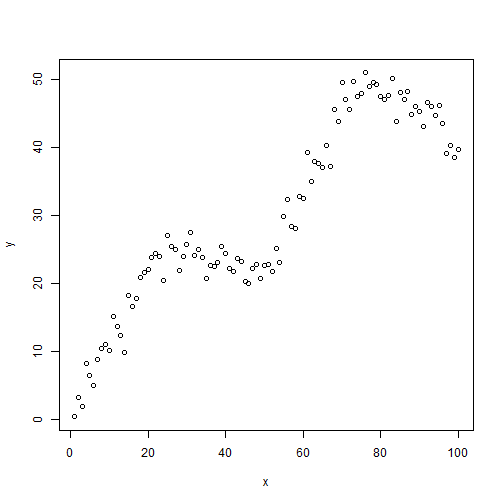It's clear there is a relationship between x and y, but the relationship is non-linear. How can we fit a linear model to this data? We could try fitting a polynomial model. The relationship seems to “change directions” four different times, so we could try fitting a 4th-degree polynomial model.

modp <- lm(y ~ poly(x, 4))
plot(x,y)
lines(x, fitted(modp))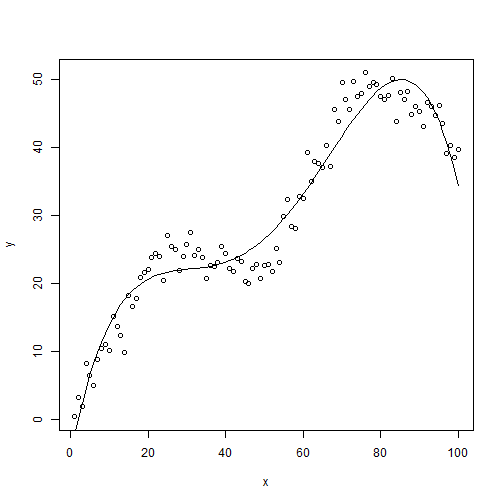This sort of captures the general nature of the relationship but the peaks and valleys just aren't quite right. They either over-predict or under-predict. We would like to do better.

Another approach might be to use a nonparametric regression approach such as loess. If we set the span parameter to 0.5, which controls the amount of smoothing, we get a decent fitting model:

modl <- loess(y ~ x, span = 0.5)
plot(x, y)
lines(x, predict(modl))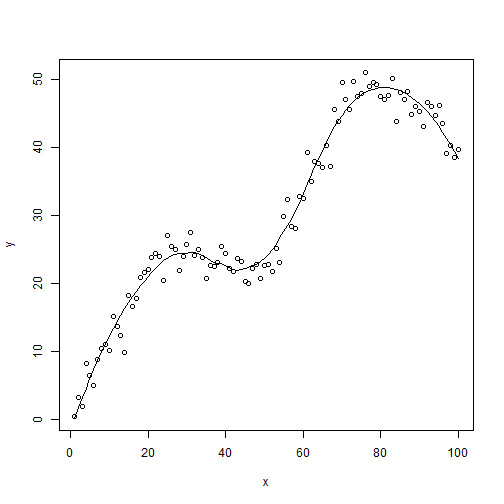This matches what we get when we use ggplot with the smooth geom:

library(ggplot2)
ggplot(data.frame(x, y), aes(x, y)) +
geom_point() +
geom_smooth(se = F, span = 0.5)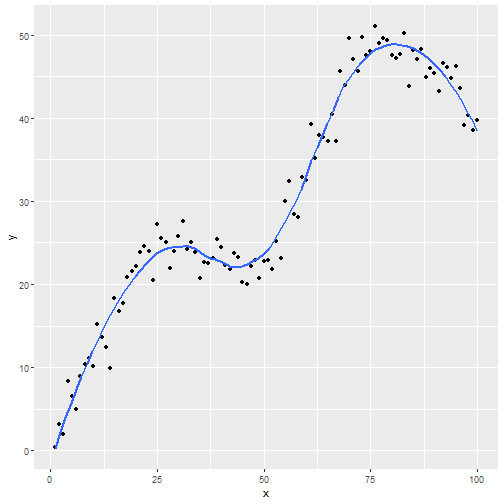But the drawback is we have no prediction equation. This is a non-parametric approach, hence no parameters were estimated.

This leads us to restricted cubic splines, or natural splines. The basic idea is to model a non-linear relationship such as the one in our example with piecewise cubic polynomials. Let's go ahead and first use natural splines in our linear model and then talk a little more about what's happening behind the scenes. Below we first load the splines package (a recommended package that comes with base R) so we have access to the ns function (natural splines). Notice we call ns on our predictor and specify df as 4. Specifying df = 4 implies 3 interior knots (ie, not including two “boundary knots”).

library(splines)
modns <- lm(y ~ ns(x, df = 4))
plot(x, y)
lines(x, predict(modns))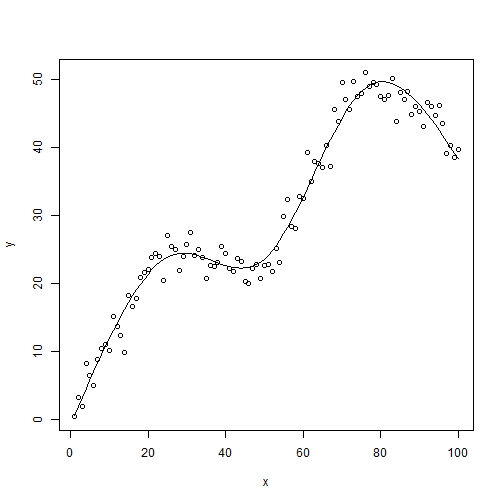This looks better than the polynomial model. And unlike the loess fit, we have a prediction equation:

summary(modns)

##
## Call:
## lm(formula = y ~ ns(x, df = 4))
##
## Residuals:
##    Min     1Q Median     3Q    Max
## -6.355 -1.302 -0.052  1.279  5.325
##
## Coefficients:
##                Estimate Std. Error t value Pr(>|t|)
## (Intercept)      0.6489     0.8242   0.787    0.433
## ns(x, df = 4)1  11.9996     1.0508  11.420   <2e-16 ***
## ns(x, df = 4)2  50.1753     1.0438  48.071   <2e-16 ***
## ns(x, df = 4)3  72.0807     2.1079  34.195   <2e-16 ***
## ns(x, df = 4)4  19.5918     0.9794  20.004   <2e-16 ***
## ---
## Signif. codes:  0 '***' 0.001 '**' 0.01 '*' 0.05 '.' 0.1 ' ' 1
##
## Residual standard error: 2.114 on 95 degrees of freedom
## Multiple R-squared:  0.977,  Adjusted R-squared:  0.976
## F-statistic:  1008 on 4 and 95 DF,  p-value: < 2.2e-16


Obviously the coefficients defy interpretation, but we can work with them as we would any other linear model. For example, we can test for linearity, that is $$H_0: \beta_2 = \beta_3 = \beta_4 = 0$$. In our model that means testing that the last 3 coefficients are equal to 0. The car package provides the powerful linearHypothesis function for this purpose.

library(car)
linearHypothesis(modns, names(coef(modns))[3:5])

## Linear hypothesis test
##
## Hypothesis:
## ns(x, df = 4)2 = 0
## ns(x, df = 4)3 = 0
## ns(x, df = 4)4 = 0
##
## Model 1: restricted model
## Model 2: y ~ ns(x, df = 4)
##
##   Res.Df     RSS Df Sum of Sq      F    Pr(>F)
## 1     98 18311.8
## 2     95   424.4  3     17887 1334.7 < 2.2e-16 ***
## ---
## Signif. codes:  0 '***' 0.001 '**' 0.01 '*' 0.05 '.' 0.1 ' ' 1


It's no surprise that the test is highly significant. We can also verify our model with natural splines is superior to the polynomial model via AIC. (Recall a lower AIC is better.)

AIC(modp, modns)

##       df      AIC
## modp   6 521.2002
## modns  6 440.3375


Now that we have played with natural splines, let's back up and try to get a better understanding of what's going on. To begin with, here's how we generated the data:

x <- 1:100         # independent variable
k <- c(25, 50, 75) # 3 interior knots

# function to construct variables x2, x3, x4
u <- function(x)ifelse(x > 0, x, 0)

x2 <- u(x - k)
x3 <- u(x - k)
x4 <- u(x - k)

# generate data
set.seed(1)
y <- 0.8 + 1*x + -1.2*x2 + 1.4*x3 + -1.6*x4 + rnorm(100,sd = 2.2)
plot(x, y)Our first predictor is x, which is simply the numbers 1 – 100. Next we define 3 “knots” at 25, 50, and 75. We then use those knots to construct three additional variables: x2, x3, and x4.

• x2 is equal to x – 25 when x – 25 is greater than 0
• x3 is equal to x – 50 when x – 50 is greater than 0
• x4 is equal to x – 75 when x – 75 is greater than 0

Finally we generate our dependent variable, y, as a function of x, x2, x3, and x4 plus some noise from a Normal(0, 2.2) distribution. The formula on the right side of the assignment operator is our True model. This is technically called a linear spline function. The best linear model we could fit to the data would be the following:

mod <- lm(y ~ x + x2 + x3 + x4)
summary(mod)

##
## Call:
## lm(formula = y ~ x + x2 + x3 + x4)
##
## Residuals:
##     Min      1Q  Median      3Q     Max
## -5.0699 -1.2797  0.1007  1.2322  4.9155
##
## Coefficients:
##             Estimate Std. Error t value Pr(>|t|)
## (Intercept)  1.34673    0.77485   1.738   0.0854 .
## x            0.97506    0.04425  22.035   <2e-16 ***
## x2          -1.14818    0.07094 -16.186   <2e-16 ***
## x3           1.35246    0.06220  21.743   <2e-16 ***
## x4          -1.57494    0.06865 -22.941   <2e-16 ***
## ---
## Signif. codes:  0 '***' 0.001 '**' 0.01 '*' 0.05 '.' 0.1 ' ' 1
##
## Residual standard error: 2.01 on 95 degrees of freedom
## Multiple R-squared:  0.9792, Adjusted R-squared:  0.9783
## F-statistic:  1117 on 4 and 95 DF,  p-value: < 2.2e-16

plot(x, y)
lines(x, fitted(mod))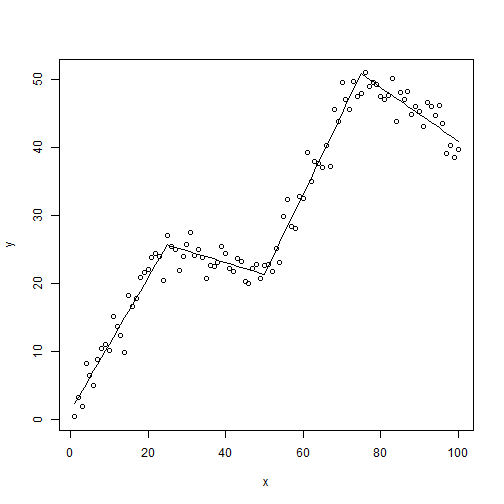So we see that to make the trajectory of our data change directions 4 times, we needed to create 4 predictors, 3 of which were based on one. This might make intuitive sense if we think of a simple parabola. It changes directions twice and has two coefficients for the $$x$$ and $$x^2$$ parameters. It's a 2nd degree polynomial. Likewise for a 3rd degree polynomial, a 4th degree polynomial, and so forth. There's only one $$x$$, but the trajectory of $$y$$ changes depending on the degree of the polynomial.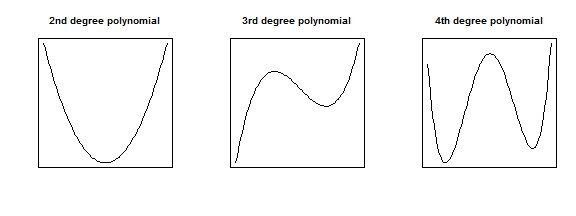The takeaway is that when we see that our response variable has a non-linear relationship with a predictor variable in real life, we may need to consider more than just a single slope coefficient to model the relationship. In the example we've been using the slope, or trajectory, changes directions 4 times, which suggests using four predictors instead of one. We tried a 4th degree polynomial of $$x$$ but saw that didn't work as well as we would have liked. A recommended approach then is to try natural splines.

The basic, and I mean very basic, idea of natural splines is to fit a 3rd degree polynomial to data within knots, and then connect those lines together. For example, below is our data with knots defined at 0, 25, 50, 75, and 100.

plot(x,y)
abline(v = c(0,25,50,75,100))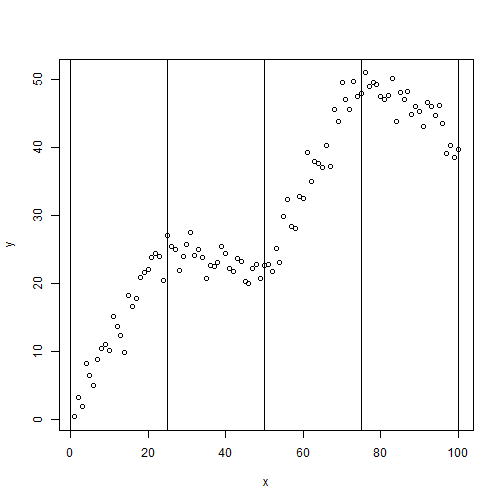With 5 knots, we have 4 regions of data. Within those 4 regions of data, natural splines essentially allow us to fit 4 different 3rd degree polynomials, all smoothed together. The magic is in how the 3 additional predictors are generated. Using the ns function in the splines package, we can create a basis matrix that allows us to fit a natural cubic spline using regular regression functions such as lm and glm. How the basis matrix is generated is quite complicated and probably something you'll just want to take on faith, like I do.

We can sort of see the natural spline in action if we fit and then color the lines between the knots. Below we regress $$y$$ on a natural spline of $$x$$ with knots defined at 25, 50 and 70. We then color the fitted lines differently between the knots.

plot(x,y)
mod.ns <- lm(y ~ ns(x, knots = c(25,50,75)))
lines(x[1:25], fitted(mod.ns)[1:25],col=1)
lines(x[26:50], fitted(mod.ns)[26:50],col=2)
lines(x[51:75], fitted(mod.ns)[51:75],col=3)
lines(x[76:100], fitted(mod.ns)[76:100],col=4)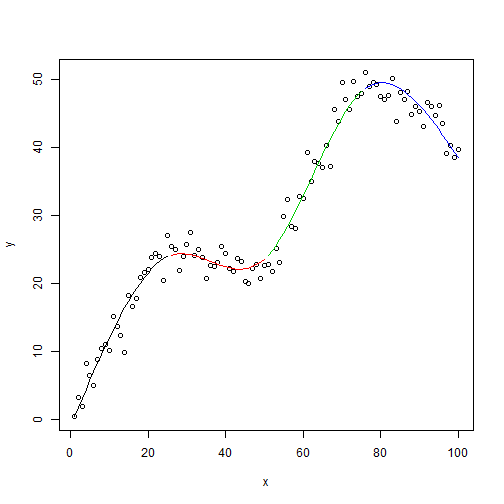Notice the red and green interior lines have a noticeable 3rd degree polynomial shape. The exterior red and blue lines look more like a 2nd degree polynomial. That's because natural splines are constrained to be linear in the tails (ie, the boundary knots). For this reason, natural splines are sometimes called restricted cubic splines. If we didn't want that constraint, we could use the bs function to generate a b-spline matrix basis. Here's what that looks like.

plot(x,y)
mod.bs <- lm(y ~ bs(x, knots = c(25,50,75)))
lines(x[1:25], fitted(mod.bs)[1:25],col=1)
lines(x[26:50], fitted(mod.bs)[26:50],col=2)
lines(x[51:75], fitted(mod.bs)[51:75],col=3)
lines(x[76:100], fitted(mod.bs)[76:100],col=4)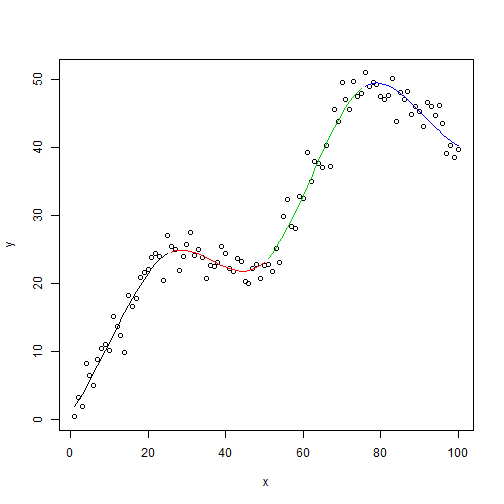Notice the blue line in particular curls up at the boundary as would be expected of a 3rd degree polynomial. But that's not the only difference. Look at the summary output. Notice the bs function generated matrix with 6 columns instead of 4.

summary(mod.bs)

##
## Call:
## lm(formula = y ~ bs(x, knots = c(25, 50, 75)))
##
## Residuals:
##     Min      1Q  Median      3Q     Max
## -5.8247 -1.1582 -0.0468  1.2780  5.0283
##
## Coefficients:
##                               Estimate Std. Error t value Pr(>|t|)
## (Intercept)                      1.983      1.218   1.628  0.10699
## bs(x, knots = c(25, 50, 75))1    6.623      2.281   2.903  0.00461 **
## bs(x, knots = c(25, 50, 75))2   33.328      1.529  21.794  < 2e-16 ***
## bs(x, knots = c(25, 50, 75))3    8.281      1.840   4.501 1.96e-05 ***
## bs(x, knots = c(25, 50, 75))4   60.284      1.722  35.000  < 2e-16 ***
## bs(x, knots = c(25, 50, 75))5   40.697      1.913  21.278  < 2e-16 ***
## bs(x, knots = c(25, 50, 75))6   38.377      1.688  22.736  < 2e-16 ***
## ---
## Signif. codes:  0 '***' 0.001 '**' 0.01 '*' 0.05 '.' 0.1 ' ' 1
##
## Residual standard error: 2.052 on 93 degrees of freedom
## Multiple R-squared:  0.9788, Adjusted R-squared:  0.9774
## F-statistic: 714.2 on 6 and 93 DF,  p-value: < 2.2e-16


So fitting a b-spline means actually fitting a more complicated model. For this reason and the fact that b-splines can be poory behaved in the tails (Harrell, p. 24), most statisticians recommend working with natural splines.

To wrap up, let's go over some guidelines for using natural splines with real data. First off, you don't want to go looking at your data and guessing how many times it changes direction to determine your knots or degrees of freedom! I did that simply as a way to help explain how splines worked. In practice you'll want to determine degrees of freedom based on sample size and how important you suspect a predictor to be. Harrell states that 4 degrees of freedom is usually sufficient. If your sample is on the small side, perhaps choose 3 degrees of freedom. If it's large, go with 5. And notice we're talking about degrees of freedom, not knots. The location of knots isn't that crucial and ns will automatically select knots based on the quantiles of the predictor.

Something else to remember is that the coefficients on a model with natural splines defy any sort of interpretation. So forget using the “1-unit increase in x leads to a __ increase in y” method to explain association. An alternative approach is an effect plot, which allows you to visualize your model given certain predictor values. Here's a quick demonstration using a simplified example that comes with the powerful effects package. Below we model the log of prestige, a prestige score for someone's occupation, as a function of logged income and a 4 degree of freedom natural spline basis of education. Calling summary and anova on the model object will reveal the natural spline appears warranted and highly significant.

library(effects)
mod.pres1 <- lm(log(prestige) ~ log(income) + ns(education, 4),
data=Prestige)


But what does it mean? What is the association between prestige and education when, say, holding income at the mean value? An effect plot sheds some light.

eff.log <- Effect("education", mod.pres1, transformation=list(inverse=exp))
plot(eff.log)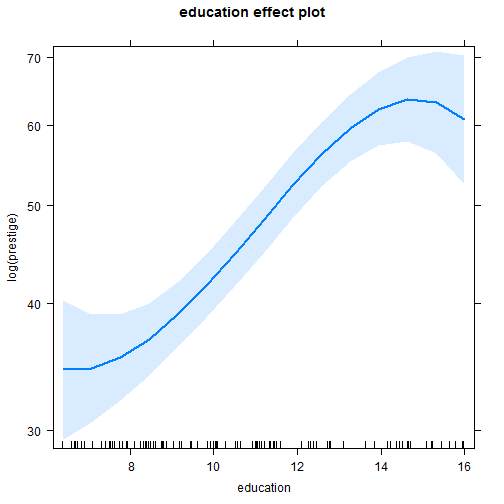This shows that from about 9 – 14, the effect of education is pretty dramatic on prestige scores, but rather uncertain in the extremes, below 9 and above 14. From 10 – 14, it looks like a 2-level increase in education is worth about a 10 point increase in prestige scores. We couldn't guess that from the summary output but we can sort of infer it from the effect plot. Again, this is just an example and not a replication of the original analysis.

Reference:

F. Harrell. Regression Modeling Strategies: With Applications to Linear Models, Logistic and Ordinal Regression, and Survival Analysis. 2nd Ed Springer. 2015

# Recreating a Geometric CDF plot from Casella and Berger

For reasons I no longer remember I decided a while back to reproduce Figure 1.5.2 from Casella and Berger (page 32):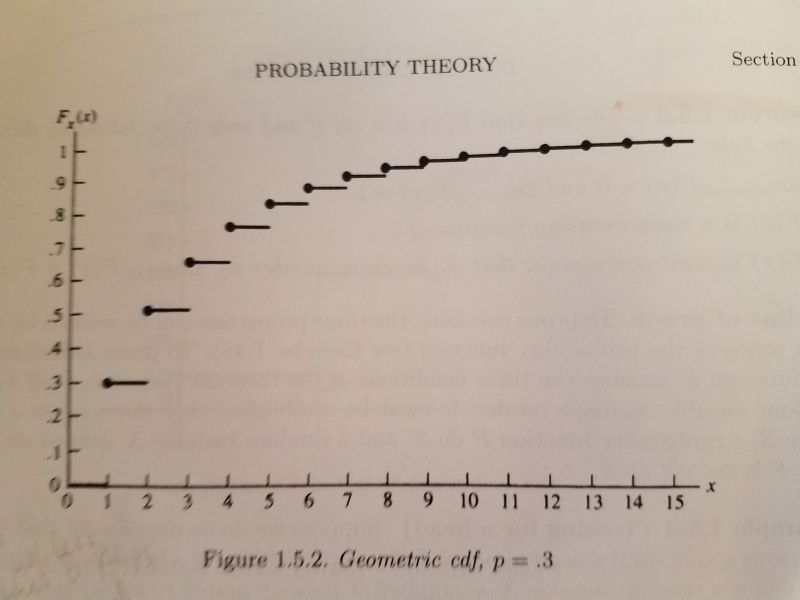It's a plot of the cumulative distribution function of a geometric distribution with p = 0.3. The geometric distribution is best explained with coin-flipping. Let's say we keep flipping a coin until we observe a head. Now let X be a random variable that equals the number of tosses required to see a head. So if we get a head on the first flip of the coin, that means we needed one toss. If it takes two tosses, we got a tail on the first toss and then a head on the second toss. The graph above visualizes the possibilities for 15 flips with probability of getting heads set to 0.3. We see that the probability of taking only one toss is 0.3, which makes sense since the probability of getting heads is 0.3. The probability of requiring two tosses is about 0.5. And so on. The straight lines indicate the probabilities only change at the whole numbers. There is no such thing as, say, 2.23 flips. This is often referred to as a step function. We see that there's a high probability of getting a head by the 5th or 6th flip. This is a simple waiting-time distribution that can be used to model the number of successes before a failure and vice-versa.

To recreate this plot in R I used the pgeom function, which returns the cumulative probability of the geometric distribution for a given number of failures before a success occurs and a given probability of success. For example, the probability of 0 failures before observing a success with p = 0.3:

pgeom(0, prob = 0.3)

##  0.3


The probability of 1 failure before observing a success with p = 0.3:

pgeom(1, prob = 0.3)

##  0.51


To generate all the probabilities in the plot, we can do the following:

y <- pgeom(q = 0:14, prob = 0.3)


Notice that instead of using 1:15 (number of total flips for success), we use 0:14. That's because pgeom works with the number of failures before success. Now that we have our y coordinates, we can create our first version of the plot as follows:

plot(x = 1:15, y, pch = 19)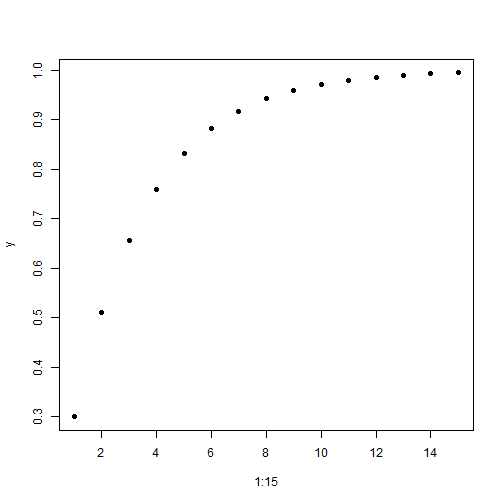Now let's add the horizontal lines using the segments function:

plot(x = 1:15, y, pch = 19)
segments(x0 = 0:15, y0 = c(0, y),
x1 = 1:16, y1 = c(0, y), lwd = 2)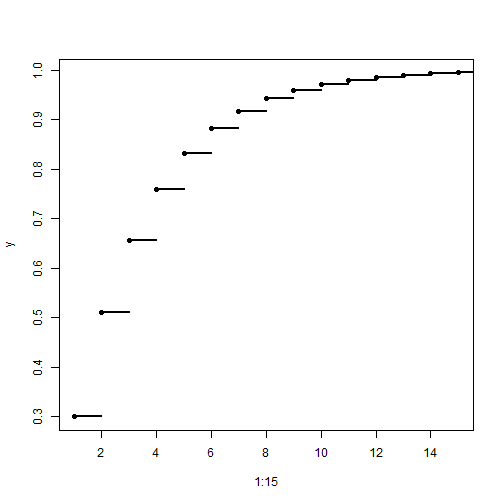The x0 and y0 coordinates are where the line starts. The x1 and y1 coordinates are where the line ends. Since the lines are horizontal, the y coordinates are the same for the start and end postions. The y coordinates include 0, so we add that value to y with the c function. The lwd = 2 argument makes the line a little thicker and darker.

Our plot is basically done, but just for fun I wanted to see how close I could make it look like the version in the book. That means relabeling the axes, moving the axis labels to the ends, and removing the lines at the top and right side of the plot. It also means moving the axis tick marks inside the plotting area. After some trial and error and deep dives into R documentation, here's what I was able to come up with:

plot(x = 1:15, y, pch = 19,
yaxt = "n", xaxt = "n", ylim = c(0,1.05), xlim = c(0,15.5),
bty="l", xaxs="i", yaxs = "i", xlab = "", ylab = "")
segments(x0 = 0:15, y0 = c(0, y),
x1 = 1:16, y1 = c(0, y), lwd = 2)
axis(side = 1, at = 0:15,
labels = 0:15, tcl = 0.5, family = "serif")
axis(side = 2, at = seq(0,1,0.1),
labels = c(0,paste(".",1:9),1), las=1, tcl = 0.5, family = "serif")
mtext(text = expression(italic(x)), side = 4,
at = 0, las = 1, line = 0.5, family = "serif")
mtext(text = expression(italic(F[x](x))), side = 3,
at = 0, line = 0.5, family = "serif")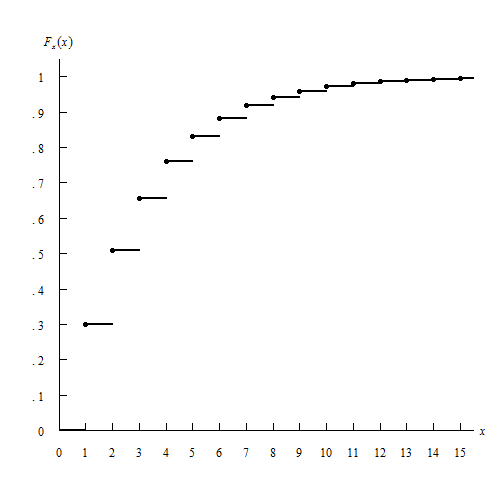In the plot function:

• the yaxt = "n" and xaxt = "n" arguments say “don't label the axes”. I instead use the axis function to create the axes.
• the ylim = c(0,1.05) and xlim = c(0,15.5) arguments tell the axes to end at 1.05 and 15.5, respectively. I wanted them to extend beyond the last value just as they do in the book.
• the bty="l" argument says “draw a box around the plot like a capital letter L”
• the xaxs="i" and yaxs = "i" arguments ensures the axes fit within the original range of the data. The default is to extend the range by 4 percent at each end. Again, I was trying to recreate the graph in the book. Notice how the origin has the x-axis and y-axis 0 values right next to one another.
• The xlab = "" and ylab = "" set blank axis labels. I instead use the mtext function to add axis labels.

The segments function remained unchanged.

The axis function allows us to explicitly define how the axis is created.

• The side argument specifies which side of the plot we're placing the axis. 1 is the bottom, 2 is the left.
• at is where we draw the tick marks.
• labels are how we label the tick marks.
• The tcl argument specifies how long to make the tick marks and in what direction. A positive value extends the tick marks into the plotting region.
• The las argument in the second axis function makes the labels on the y-axis horizontal.

Finally I used the mtext function to create the axis labels. mtext writes text into the margins of a plot and can take some trial and error to get the placement of text just so.

• The text argument is what text we want to place in the graph. In this case I make use of the expression function which allows us to create mathematical notation. For example, the syntax expression(italic(F[x](x))) returns $$F_x (x)$$
• The side argument again refers to where in the plot to place the text. 3 is top and 4 is right. This means the y-axis label is actually in the top of the plot and the x-axis label is on the right. A little bit of a hack.
• at says where to place the text along the axis parallel to the margin. In both cases we use 0. We want the y-axis label at the 0 point corresponding to the x-axis, and the x-axis label at the 0 point corresponding to the y-axis. A little confusing, I think.
• The las argument rotates the x label to be horizontal
• The line argument specifies on which margin line to place the text, starting at 0 counting outwards. This is one that takes some trial and error to get just right.
• The family argument specifies the type of font to use. “serif” is like Times New Roman.

Not perfect, but close enough. Of course I much prefer the R defaults when it comes to plotting layout. Even though R allows us to recreate this graph, I don't think it's necessarily a “good” graph.

I also decided to tackle this using ggplot. Here's how far I got.

library(ggplot2)
dat <- data.frame(x = 1:15, y = pgeom(q = 0:14, prob = 0.3))
dat2 <- data.frame(x = 0:15, y = c(0, dat$y[-16]), xend = 1:16, yend = c(0,dat$y[-16]))

ggplot(dat, aes(x = x, y = y)) + geom_point() +
geom_segment(aes(x = x, y = y, xend = xend, yend = yend), data = dat2) +
scale_x_continuous(breaks = 0:15, labels = 0:15) +
scale_y_continuous(breaks = seq(0,1,0.1), labels = c(0,paste(".",1:9),1)) +
labs(x = "x", y = expression(italic(F[x](x)))) +
theme(panel.background = element_blank(),
axis.line.x = element_line(color = "black"),
axis.line.y = element_line(color = "black"),
axis.title.x = element_text(face = "italic", hjust = 1),
axis.title.y = element_text(face = "italic", hjust = 1, angle = 0))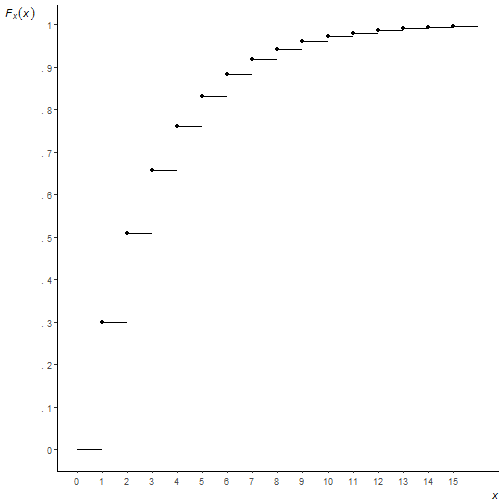You can see I couldn't figure out how to move the axis ticks into the plotting region, or how to place axis labels at the ends of the axis, or how to get the origin to start at precisely (0, 0). I'm not saying it can't be done, just that I lost interest in trying to go further. And a very strong argument can be made that these are things you shouldn't do anyway! But as I said at the outset, this was all for fun.

# Revisiting some old R-code

One of my first blog posts on this site simulated rolling a die 6 times and observing if side i was observed on the ith roll at least once. (For example, rolling a 3 on my 3rd roll would be a success.) I got the idea from one of my statistics textbooks which had a nice picture of the simulation converging to the true probability of 0.665 over the course of 500 trials. I was able to recreate the simulation in R and I guess I got excited and blogged about it. I was reminded of this today when I opened that old textbook and came across the R code that I had actually written in the margin by hand. Apparently I was super proud of my ground-breaking R code and decided to preserve it for posterity in writing. smh

Over 5 years later my precious R code looks pretty juvenile. It’s way more complicated than it needs to be and doesn’t take advantage of R's vectorized calculations. As Venables and Ripley say in MASS, “Users coming to S from other languages are often slow to take advantage of the power of S to do vectorized calculations…This often leads to unnecessary loops.” Indeed. I'm living proof of that.

I shouldn’t be too hard on my myself though. I was not yet familiar with functions like replicate and cumsum. And I was more focused on recreating the plot than writing optimal R code. I went with what I knew. And R, so forgiving and flexible, accommodated my novice enthusiasm.

Here is how I would approach the problem today:

r.out <- replicate(n = 500, any(sample(1:6, size = 6, replace = T) == 1:6))
p.out <- cumsum(r.out)/seq(500)
plot(x = seq(500), y = p.out, type = "l", ylim = c(0,1),
main = "Convergence to probability as n increases", xlab = "n")
abline(h = 0.665)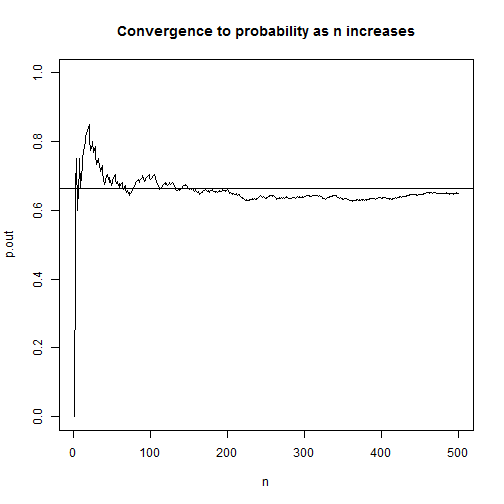On line 1, we use the sample function to “roll a die 6 times” by sampling the numbers 1 – 6, with replacement, 6 times. Then we compare the 6 results with the vector of numbers 1 – 6 using the == operator and use the any function to check if any are TRUE. Next we replicate that 500 times and store the result in r.out. This is a vector of TRUE/FALSE values which R treats numerically as 1 and 0. This means we can use cumsum to find the cumulative sum of successes. To determine the cumulative proportion of successes, we divide each cumulative sum by the trial number. The result is a vector of proportions that should start converging to 0.665. Finally we plot using base R plot and abline.

This is more efficient than my original attempt 5 years ago and better captures the spirit of the simulation. I'm sure 5 years from now if I stumble upon this post I'll have yet another more elegant way to do it. I'm already looking at it thinking, “I should have generalized this with a function, and used ggplot2 to make the graph. And I shouldn't do seq(500) twice.” In fact I know I could have avoided the replicate function by using the fact that there's a probability of $$\frac{1}{6}$$ of observing side i on the ith roll of a die. So I could have used a single rbinom call to do the simulation, like so:

r.out2 <- cumsum(rbinom(n = 500, size = 6, prob = 1/6) > 0)
p.out2 <- r.out2/seq(500)
plot(x = seq(500), y = p.out2, type = "l", ylim = c(0,1),
main = "Convergence to probability as n increases", xlab = "n")
abline(h = 0.665)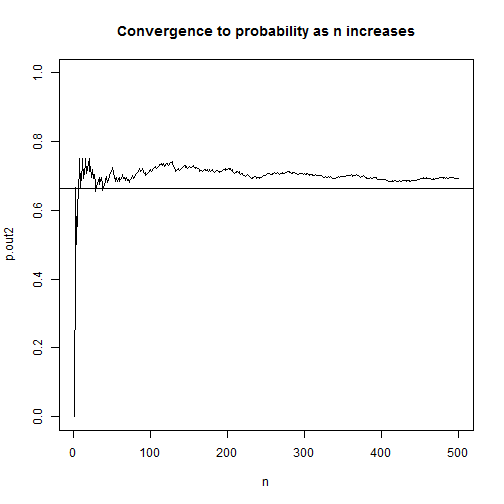In this version instead of simulating 6 literal die rolls, we simulate the number of successes in 6 die rolls. We turn each roll of the die into a binomial event: success or failure. The rbinom function allows us to simulate binomial events where size is the number of trials (or rolls in this case) and prob is the probability of success at each trial. So rbinom(n = 1, size = 6, prob = 1/6) would return a number ranging 0 to 6 indicating the number of success. Think of it as flipping 6 coins, each with probability of getting heads as $$\frac{1}{6}$$, and then counting the number of heads we observed. Setting the n argument to 500 replicates it 500 times. After that it's simply a matter of logically checking which outcomes were greater than 0 and using cumsum on the resulting TRUE/FALSE vector.

This version is way faster. I mean way faster. Compare the time it takes it to do each 1,000,000 times:

system.time({
r.out <- replicate(n = 1e6, any(sample(1:6, size = 6, replace = T) == 1:6))
p.out <- cumsum(r.out)/seq(1e6)
})

##    user  system elapsed
##    5.26    0.00    5.26

system.time({
r.out2 <- cumsum(rbinom(n = 1e6, size = 6, prob = (1/6)) > 0)
p.out2 <- r.out2/seq(1e6)
})

##    user  system elapsed
##    0.06    0.00    0.06


It's not even close. Who was the dummy that wrote that first version with replicate?

But does the new faster version reflect the experimental setting better? Not really. Remember, we're demonstrating probability concepts with die rolls in the first chapter of an intro stats textbook. That's probably not the best time to break out rbinom. And the demo was for 500 trials, not 1,000,000. I had to ramp up the trials to see the speed difference. Maybe the “right” R code in this situation is not the fastest version but rather the one that's easier to understand.

# Profile likelihood ratio confidence intervals

When you fit a generalized linear model (GLM) in R and call confint on the model object, you get confidence intervals for the model coefficients. But you also get an interesting message:

Waiting for profiling to be done...

What's that all about? What exactly is being profiled? Put simply, it's telling you that it's calculating a profile likelihood ratio confidence interval.

The typical way to calculate a 95% confidence interval is to multiply the standard error of an estimate by some normal quantile such as 1.96 and add/subtract that product to/from the estimate to get an interval. In the context of GLMs, we sometimes call that a Wald confidence interval.

Another way to determine an upper and lower bound of plausible values for a model coefficient is to find the minimum and maximum value of the set of all coefficients that satisfy the following:

$-2\log\left(\frac{L(\beta_{0}, \beta_{1}|y_{1},…,y_{n})}{L(\hat{\beta_{0}}, \hat{\beta_{1}}|y_{1},…,y_{n})}\right) < \chi_{1,1-\alpha}^{2}$

Inside the parentheses is a ratio of likelihoods. In the denominator is the likelihood of the model we fit. In the numerator is the likelihood of the same model but with different coefficients. (More on that in a moment.) We take the log of the ratio and multiply by -2. This gives us a likelihood ratio test (LRT) statistic. This statistic is typically used to test whether a coefficient is equal to some value, such as 0, with the null likelihood in the numerator (model without coefficient, that is, equal to 0) and the alternative or estimated likelihood in the denominator (model with coefficient). If the LRT statistic is less than $$\chi_{1,0.95}^{2} \approx 3.84$$, we fail to reject the null. The coefficient is statisically not much different from 0. That means the likelihood ratio is close to 1. The likelihood of the model without the coefficient is almost as high the model with it. On the other hand, if the ratio is small, that means the likelihood of the model without the coefficient is much smaller than the likelihood of the model with the coefficient. This leads to a larger LRT statistic since it's being log transformed, which leads to a value larger than 3.84 and thus rejection of the null.

Now in the formula above, we are seeking all such coefficients in the numerator that would make it a true statement. You might say we're “profiling” many different null values and their respective LRT test statistics. Do they fit the profile of a plausible coefficient value in our model? The smallest value we can get without violating the condition becomes our lower bound, and likewise with the largest value. When we're done we'll have a range of plausible values for our model coefficient that gives us some indication of the uncertainly of our estimate.

Let's load some data and fit a binomial GLM to illustrate these concepts. The following R code comes from the help page for confint.glm. This is an example from the classic Modern Applied Statistics with S. ldose is a dosing level and sex is self-explanatory. SF is number of successes and failures, where success is number of dead worms. We're interested in learning about the effects of dosing level and sex on number of worms killed. Presumably this worm is a pest of some sort.

# example from Venables and Ripley (2002, pp. 190-2.)
ldose <- rep(0:5, 2)
numdead <- c(1, 4, 9, 13, 18, 20, 0, 2, 6, 10, 12, 16)
sex <- factor(rep(c("M", "F"), c(6, 6)))
budworm.lg <- glm(SF ~ sex + ldose, family = binomial)
summary(budworm.lg)

##
## Call:
## glm(formula = SF ~ sex + ldose, family = binomial)
##
## Deviance Residuals:
##      Min        1Q    Median        3Q       Max
## -1.10540  -0.65343  -0.02225   0.48471   1.42944
##
## Coefficients:
##             Estimate Std. Error z value Pr(>|z|)
## (Intercept)  -3.4732     0.4685  -7.413 1.23e-13 ***
## sexM          1.1007     0.3558   3.093  0.00198 **
## ldose         1.0642     0.1311   8.119 4.70e-16 ***
## ---
## Signif. codes:  0 '***' 0.001 '**' 0.01 '*' 0.05 '.' 0.1 ' ' 1
##
## (Dispersion parameter for binomial family taken to be 1)
##
##     Null deviance: 124.8756  on 11  degrees of freedom
## Residual deviance:   6.7571  on  9  degrees of freedom
## AIC: 42.867
##
## Number of Fisher Scoring iterations: 4


The coefficient for ldose looks significant. Let's determine a confidence interval for the coefficient using the confint function. We call confint on our model object, budworm.lg and use the parm argument to specify that we only want to do it for ldose:

confint(budworm.lg, parm = "ldose")

## Waiting for profiling to be done...

##     2.5 %    97.5 %
## 0.8228708 1.3390581


We get our “waiting” message though there really was no wait. If we fit a larger model and request multiple confidence intervals, then there might actually be a waiting period of a few seconds. The lower bound is about 0.8 and the upper bound about 1.32. We might say every increase in dosing level increase the log odds of killing worms by at least 0.8. We could also exponentiate to get a CI for an odds ratio estimate:

exp(confint(budworm.lg, parm = "ldose"))

## Waiting for profiling to be done...

##    2.5 %   97.5 %
## 2.277027 3.815448


The odds of “success” (killing worms) is at least 2.3 times higher at one dosing level versus the next lower dosing level.

To better understand the profile likelihood ratio confidence interval, let's do it “manually”. Recall the denominator in the formula above was the likelihood of our fitted model. We can extract that with the logLik function:

den <- logLik(budworm.lg)
den

## 'log Lik.' -18.43373 (df=3)


The numerator was the likelihood of a model with a different coefficient. Here's the likelihood of a model with a coefficient of 1.05:

num <- logLik(glm(SF ~ sex + offset(1.05*ldose), family = binomial))
num

## 'log Lik.' -18.43965 (df=2)


Notice we used the offset function. That allows us to fix the coefficient to 1.05 and not have it estimated.

Since we already extracted the log likelihoods, we need to subtract them. Remember this rule from algebra?

$\log\frac{M}{N} = \log M – \log N$

So we subtract the denominator from the numerator, multiply by -2, and check if it's less than 3.84, which we calculate with qchisq(p = 0.95, df = 1)

-2*(num - den)

## 'log Lik.' 0.01184421 (df=2)

-2*(num - den) < qchisq(p = 0.95, df = 1)

##  TRUE


It is. 1.05 seems like a plausible value for the ldose coefficient. That makes sense since the estimated value was 1.0642. Let's try it with a larger value, like 1.5:

num <- logLik(glm(SF ~ sex + offset(1.5*ldose), family = binomial))
-2*(num - den) < qchisq(p = 0.95, df = 1)

##  FALSE


FALSE. 1.5 seems too big to be a plausible value for the ldose coefficient.

Now that we have the general idea, we can program a while loop to check different values until we exceed our threshold of 3.84.

cf <- budworm.lg$coefficients # fitted coefficient 1.0642 cut <- qchisq(p = 0.95, df = 1) # about 3.84 e <- 0.001 # increment to add to coefficient LR <- 0 # to kick start our while loop while(LR < cut){ cf <- cf + e num <- logLik(glm(SF ~ sex + offset(cf*ldose), family = binomial)) LR <- -2*(num - den) } (upper <- cf)  ## ldose ## 1.339214  To begin we save the original coefficient to cf, store the cutoff value to cut, define our increment of 0.001 as e, and set LR to an initial value of 0. In the loop we increment our coefficient estimate which is used in the offset function in the estimation step. There we extract the log likelihood and then calculate LR. If LR is less than cut (3.84), the loop starts again with a new coefficient that is 0.001 higher. We see that our upper bound of 1.339214 is very close to what we got above using confint (1.3390581). If we set e to smaller values we'll get closer. We can find the LR profile lower bound in a similar way. Instead of adding the increment we subtract it: cf <- budworm.lg$coefficients  # reset cf
LR <- 0                           # reset LR
while(LR < cut){
cf <- cf - e
num <- logLik(glm(SF ~ sex + offset(cf*ldose), family = binomial))
LR <- -2*(num - den)
}
(lower <- cf)

##    ldose
## 0.822214


The result, 0.822214, is very close to the lower bound we got from confint (0.8228708).

This is a very basic implementation of calculating a likelihood ratio confidence interval. It is only meant to give a general sense of what's happening when you see that message Waiting for profiling to be done.... I hope you found it helpful. To see how R does it, enter getAnywhere(profile.glm) in the console and inspect the code. It's not for the faint of heart.

I have to mention the book Analysis of Categorical Data with R, from which I gained a better understanding of the material in this post. The authors have kindly shared their R code at the following web site if you want to have a look: http://www.chrisbilder.com/categorical/

To see how they “manually” calculate likelihood ratio confidence intervals, go to the following R script and see the section “Examples of how to find profile likelihood ratio intervals without confint()”: http://www.chrisbilder.com/categorical/Chapter2/Placekick.R

# Earthquake data and Benford’s Law

Much has been written about Benford's Law, that weird phenonmenon where if you have a naturally occuring set of numerical data, 30% of the numbers will begin with 1, 18% will begin with 2, 12% will begin with 3, and so on. You might expect the distribution of leading digits to be uniformly distributed, but no, that just isn't the case. 60% of the time the leading digit is 1, 2, or 3. Though it may seem like some curious mathematical anomaly, Benford's Law has been used to detect fraud in elections and accounting.

In this post let's use R to verify that earthquake data obtained from the USGS does indeed follow Benford's Law. On May 28, 2016, I downloaded data for all earthquakes in the past 30 days. The data has a number of fields, including earthquake magnitude, depth of the earthquake, location, time and many others. Benford's Law says those numbers should follow a particular distribution.

The formula for Benford's Law is as follows:

$P(d) = \log_{10} \left(1+\frac{1}{d}\right)$

That says the probability that a digit d occurs as the first number is equal the log base 10 of 1 + 1/d. We can quickly generate these for d = 1 – 9 in R:

log10(1 + (1/(1:9)))

##  0.30103000 0.17609126 0.12493874 0.09691001 0.07918125 0.06694679
##  0.05799195 0.05115252 0.04575749


And we can make a quick plot as follows:

barplot(log10(1 + (1/(1:9))), names.arg = 1:9, main = "Benford's Law")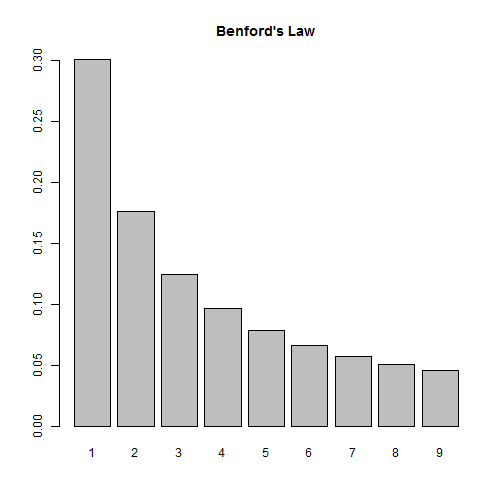So according to this law, if we look at the distribution of first digits in our earthquake data, we should see them closely follow this distribution. Let's find out!

First we need to import the data. Thanks to the USGS, this data comes ready for analysis. All we need to do is read it into R:

dat <- read.csv("all_month.csv")
nrow(dat)

##  8584


Over 8500 earthquakes in the past 30 days! A quick look at the magnitude shows us most of them are very small:

summary(dat$mag)  ## Min. 1st Qu. Median Mean 3rd Qu. Max. NA's ## -1.900 0.790 1.280 1.526 1.930 7.200 20  This also tells us we have some negative numbers (?) as well as some missing data, NA's. We also have numbers that start with 0 and have a decimal, such as 0.79. (In this case, Benford would say the number “starts” with a 7, not a 0.) This means when we determine the leading digits, we'll need to ignore negative signs, leading 0s, decimals and missing values. Let's investigate at the mag column. First we remove the NA's. Below is.na(dat$mag) generates a logical vector of TRUE and FALSE values. Adding an ! in front reverses the TRUE and FALSE values. Finally inserting the result in subsetting brackets returns only those values that are TRUE, ie not missing.

digits <- dat$mag[!is.na(dat$mag)]


Next we extract the first digits. To help with this I use two R packages, magrittr and stringr. The magrittr package allows us to “chain” commands together with the %>% operator. The stringr package provides the str_extract function that allows us to extract phrases that follow a certain pattern. So what we have below can be translated as follows:

• take the absoluet value of digits (to get rid of negative signs)
• convert digits to character (so we can use the next two functions)
• extract anything that is not a “0” or a “.” (We express this a regular expression: "[^0\\.]")
• extract the first digit (starting and ending at position 1)
library(magrittr)
library(stringr)
digits <- abs(digits) %>%
as.character() %>%
str_extract(pattern = "[^0\\.]") %>%
substr(1,1)


As an extra precaution, we then convert digits to a factor and set levels to be all digits 1 through 9. This ensure all digits are represented in subsequent calculations.

digits <- factor(digits, levels = 1:9) # ensure all digits represented


And finally we tally the first digits and calculate proportions:

table(digits) %>% prop.table()

## digits
##          1          2          3          4          5          6
## 0.42800141 0.17134139 0.05738763 0.09447248 0.05809177 0.03990142
##          7          8          9
## 0.04459570 0.05304542 0.05316277


We see 1 appearing a lot more often, but it's hard to tell how the other digits compare to Benford's Law. Let's put both distributions on the same graph. To do this, I went ahead and created a function that will allow us to check any vector of numbers against Benford's Law. Let's load the function and see how it works, then we'll break it down and explain it.

library(ggplot2)
compareBenford <- function(d){
digits <- d[!is.na(d)]
digits <- substr(stringr::str_extract(as.character(abs(digits)), pattern = "[^0\\.]"),1,1)
digits <- factor(digits, levels = 1:9) # ensure all digits represented
depth <- prop.table(table(digits))
ben <- log10(1 + (1/(1:9)))
dat2 <- data.frame(ben, depth)
names(dat2) <- c("Benford","Digit",deparse(substitute(d)))
dat2L <- reshape2::melt(dat2,id.vars="Digit", variable.name = "Type", value.name = "Frequency")
ggplot(dat2L, aes(x=Digit, y=Frequency, fill=Type)) +
geom_bar(stat = "identity", position = "dodge")
}

compareBenford(dat$mag)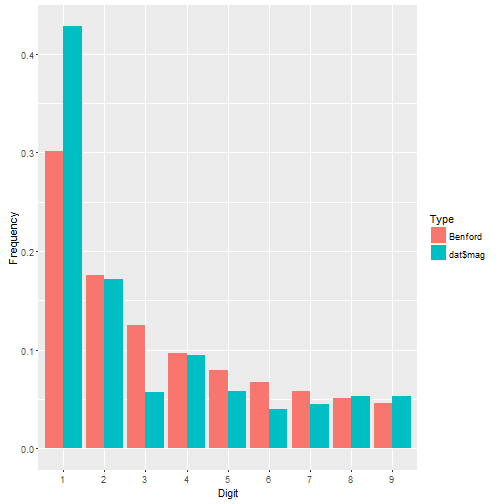We see dat$mag has more 1's than we might expect and fewer 3's, but otherwise seems to follow the distribution pretty closely. Let's check out earthquake depth.

compareBenford(dat$depth)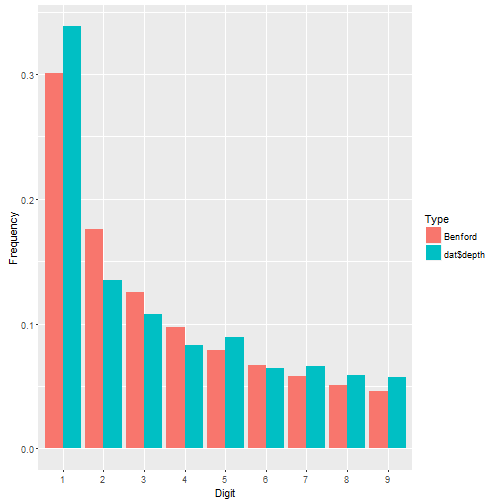This appears to fit even better. About the function: • the first four lines are what we did before. Notice I went ahead and nested the functions instead of using magrittr. That's how I originally coded it before deciding to write a blog post. Then I decided to break out magrittr for the fun of it. • After that I calculate Benford's proportions. • Then I put both sets of proportions in a data frame. • Then I change the names of the data frame. Notice there are three names to change, not two. Why? The depth vector is actually a table. When it gets pulled into a data frame, two columns are produced: the table cells and the table names. The names are the digits 1 – 9. Notice I also use deparse(substitute(d)) to name the first-digit proportions in the data frame. This ensures that whatever vector I give my function, the name of it will be the vector itself. So if I give it dat$mag, the column name of the first-digit proportions will be dat$mag. • Next I reshape the data using the melt function in the reshape2 package. This puts all the proportions in one column called Frequency and the type of proportion in another column called Type. I still have my Digit column that indicates which proportion goes with which digit. Having my data in this form allows me to use ggplot to map fill color to Type. • Finally I create the graph. I set Digit on the x-axis, Frequency on the y-axis, and map Type to the fill color. The geom_bar function says draw a bar plot. The stat = "identity" and position = "dodge" arguments say set the height of the bars to the actual values and “dodge” them so they're next to one another. Let's look at ALL numeric columns in the earthquakes data. We need to identify all numeric columns and pull them into a vector for our function to work. Here's how I do it: theCols <- sapply(dat, class) %in% c("numeric","integer") numCols <- names(dat)[theCols] allDigits <- unlist(dat[,numCols]) compareBenford(allDigits)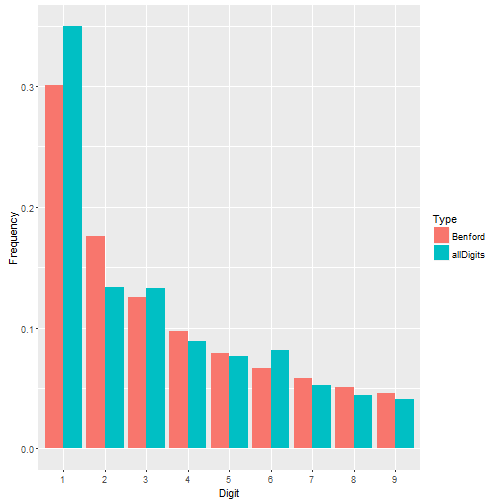Not a bad fit. Benford's Law seems to work just fine for the earthquake data. But what about data where Benford's Law doesn't work? Recall that Benford's Law applies to most naturally occuring sets of numbers. Not all. Take the iris data that come with R. These are measurements of 50 flowers from each of 3 species of iris. This is a small data set looking at very specific measurements. It doesn't seem to follow Benford's Law very well. irisDigits <- unlist(iris[,1:4]) compareBenford(irisDigits)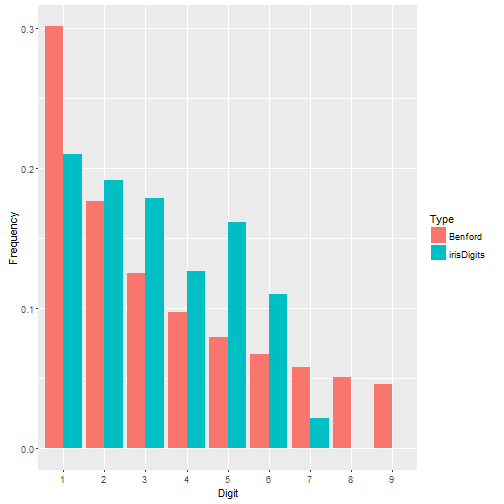# Recreating a graph from a NCHS data brief I was reading this sobering article about the rise of suicide rates in the United States and decided to read the NCHS data brief on which it was based. I noticed the source data was freely available online through the CDC Wonder databases, specifically the Detailed Mortality database, and figured I would take a look. One graph that caught my eye was Figure 2, which showed that suicide rates for females were higher in 2014 than in 1999 for all age groups under 75 years.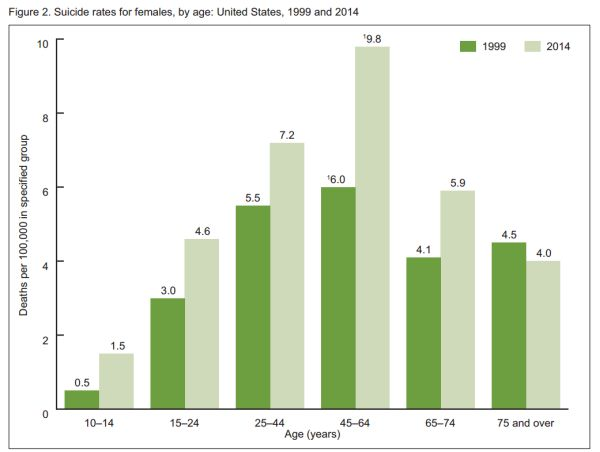That's a very sad and concerning graph. What happened in the last 15 years? I have no theories about the increase in suicide rates, but I do admire the CDC statisticians who tackle this kind of gut-wrenching data, day in and day out. Let's join them for a moment and recreate this graph in R. First we need to get the data from the Detailed Mortality database. Instead of directly downloading raw data, we submit a query for the data we want. There is a 7-step form to complete. Step 1: Group by ICD-10 113 Cause List, Cause of death, Age Groups, Gender, and Year. This uses all available pull-down lists. Step 2: leave as is. (ie, all US states) Step 3: leave as is. (ie, all genders, ages, and races) Step 4: select 1999 and 2014 using Ctrl + Click to select both years Step 5: leave as is. (ie, all weekdays, autopsies, and places of death) Step 6: select the ICD-10 113 Cause List radio button and select “Intentional self-harm (suicide) (*U03,X60-X84,Y87.0)” Step 7: click Send. The results should appear in your web browser. If everything looks good, click the Export button to download a tab-delimited file of the results. It will have a .txt extenstion. Now that we have our data, we can go to R and get to work. Before we read in the data we should open it in a text editor and see what we got. We have a header row, so that's good. If we scroll down, we'll see there's about 70 lines of metadata. This is data about our data, such as where it came from, when we downloaded it, etc. We don't want that in our data frame, but we should save it with our data frame as an attribute. To start we'll use readLines to read in the data one line at a time. I chose to do this as a way to programmatically figure out where the metadata begins. I noticed it begins after a divider of three dashes (“—”), so I use grep in combination with min to find the first occurence of this divider and save a n. raw <- readLines("Underlying Cause of Death, 1999-2014 (3).txt")  ## Warning in readLines("Underlying Cause of Death, 1999-2014 (3).txt"): ## incomplete final line found on 'Underlying Cause of Death, 1999-2014 ## (3).txt'  n <- min(grep(pattern = "---",raw))  Now I can use n with the nrows argument in the read.delim function to tell it how many rows I want to read in. Notice I have to say n - 2. I have to subtract the header row and the divider row itself. I also specify “Not Applicable” and “Unreliable” as NA values. Finally, take a look at my file name. Yep, it took me a few tries to get the data I wanted. I'm not too proud to admit that. :) dat <- read.delim(file = "Underlying Cause of Death, 1999-2014 (3).txt", header = TRUE, nrows = n-2, na.strings = c("Not Applicable","Unreliable"))  Let's go ahead and save the metadata with our data frame. We can do that with the comment function. We don't need it to create the graph, but it's good practice to preserve this kind of information. To see the metadata after we save, just enter comment(dat). It's not pretty when it's printed to the console, but we have it if we need it. comment(dat) <- raw[n:length(raw)]  Now we need to clean up the data. First order of business is to reorder the age groups. By default, R brought them in as a factor and put the 15-24 age group as the first level. We want that first level to be the 5-14 age group. It's already a factor, but we reorder the levels by making the column a factor again and specifying the levels in the specified order. The original 5th level comes first and then everything else. There may be a better way of doing this, but it works. dat$Ten.Year.Age.Groups.Code <- factor(dat$Ten.Year.Age.Groups.Code, levels = levels(dat$Ten.Year.Age.Groups.Code)[c(5,1,2,3,4,6,7,8,9,10)])


We only need females, so let's subset. I make a new data frame called dat2.

dat2 <- subset(dat, Gender=="Female")


Now if we look at Figure 2 again, the x-axis has 6 age groups, but our data has 9 age groups. So we need to combine a few age groups. Specifically we need to combine the “25-34” and “35-44” groups into “25-44”, combine the “45-54” and “55-64” groups into “45-64”, and the “75-84” and “85+” into “75+”. That's not too bad. We just reset the levels.

levels(dat2$Ten.Year.Age.Groups.Code) <- c("5-14", "15-24", "25-44", "25-44", "45-64", "45-64", "65-74", "75+", "75+", "NS")  Since we have 6 age groups instead of 9, we need to combine death totals for the three new age groups. We also need to combine death totals for all the various causes of deaths. We can do that with aggregate. Below we sum Deaths by Ten.Year.Age.Groups.Code and Year. This returns a new data frame that I call dat3. (dat3 <- aggregate(Deaths ~ Ten.Year.Age.Groups.Code + Year, data=dat2, sum))  ## Ten.Year.Age.Groups.Code Year Deaths ## 1 5-14 1999 50 ## 2 15-24 1999 575 ## 3 25-44 1999 2359 ## 4 45-64 1999 1868 ## 5 65-74 1999 420 ## 6 75+ 1999 469 ## 7 5-14 2014 151 ## 8 15-24 2014 990 ## 9 25-44 2014 3018 ## 10 45-64 2014 4195 ## 11 65-74 2014 828 ## 12 75+ 2014 477 ## 13 NS 2014 1  Next I need to calculate “deaths per 100,000” for each age group. We do this by dividing Deaths by the age group population and multiplying by 100,000. I need to add the age group populations to dat3 to do this. First I need to sum the populations for my new age groups. I can use aggregate again with dat2, but I need to remove duplicate rows of age group population values before I do this. I decided to save the de-duped data frame to tmp and not overwrite dat2 tmp <- subset(dat2, select = c("Year", "Ten.Year.Age.Groups.Code", "Population")) tmp <- tmp[!duplicated(tmp),] (dat4 <- aggregate(Population ~ Ten.Year.Age.Groups.Code + Year, data=tmp, sum))  ## Ten.Year.Age.Groups.Code Year Population ## 1 5-14 1999 19908282 ## 2 15-24 1999 18860184 ## 3 25-44 1999 42614694 ## 4 45-64 1999 31011068 ## 5 65-74 1999 10125424 ## 6 75+ 1999 10371906 ## 7 5-14 2014 20161424 ## 8 15-24 2014 21456371 ## 9 25-44 2014 41900194 ## 10 45-64 2014 42789506 ## 11 65-74 2014 14049245 ## 12 75+ 2014 11842674  Now I'm ready to merge dat3 and dat4 so I have Deaths and Populations in one data frame. Before I do that I remove the row with age group of “NS” which means “Not Stated”. We don't need that row. dat3 <- subset(dat3, Ten.Year.Age.Groups.Code != "NS") dat5 <- merge(dat3,dat4,by = c("Year","Ten.Year.Age.Groups.Code"))  And finally we can calculate Rate. I decided to also sort the data frame as well. dat5$Rate <- round(dat5$Deaths/dat5$Population * 100000,1)
(dat5 <- dat5[order(dat5$Ten.Year.Age.Groups.Code, dat5$Year),])

##    Year Ten.Year.Age.Groups.Code Deaths Population Rate
## 4  1999                     5-14     50   19908282  0.3
## 10 2014                     5-14    151   20161424  0.7
## 1  1999                    15-24    575   18860184  3.0
## 7  2014                    15-24    990   21456371  4.6
## 2  1999                    25-44   2359   42614694  5.5
## 8  2014                    25-44   3018   41900194  7.2
## 3  1999                    45-64   1868   31011068  6.0
## 9  2014                    45-64   4195   42789506  9.8
## 5  1999                    65-74    420   10125424  4.1
## 11 2014                    65-74    828   14049245  5.9
## 6  1999                      75+    469   10371906  4.5
## 12 2014                      75+    477   11842674  4.0


A quick comparison of our rates with those in Figure 2 shows that we're in agreement. Now it's time to re-create the graph. For this I chose to use ggplot2. To match the colors, I uploaded a picture of the original graph to this wonderful site and figured out the shades of green were #6D9F40 (dark green) and #CFDABA (light green).

library(ggplot2)
ggplot(dat5, aes(x=Ten.Year.Age.Groups.Code, y=Rate, fill=factor(Year))) +
geom_bar(stat="identity", position = "dodge") +
labs(x="Age (years)",y="Deaths per 100,000 in specified group") +
scale_fill_manual("Year", values = c("#6D9F40","#CFDABA")) +
annotate(geom = "text", x = (rep(1:6,each=2) + c(-1,1)*0.22),
y = dat5$Rate + 0.2, label = sprintf("%.1f", dat5$Rate)) +
theme_bw() +
scale_y_continuous(breaks=seq(0,10,2))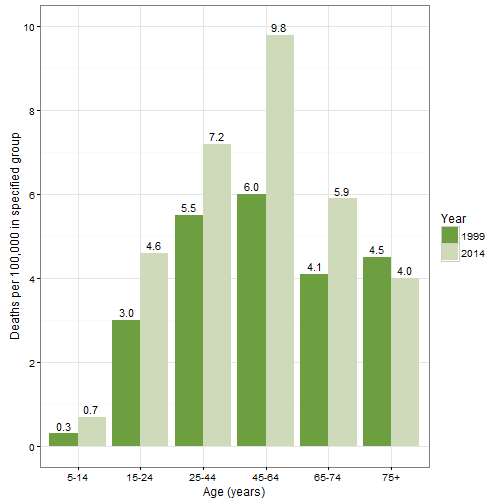The first line defines the aesthetics. I want Year to be treated like a categorical variable, so I call it with factor. The next lines says, “don't count the rows to create the bar graph, use the actual values of Rate (ie, identity).” It also says “dodge”“ the Years so they're next to each other (instead of stacked). The third line labels the axes. The fourth line titles the legend and defines the colors. The annontate line adds the counts to the plot. That took some trial and error! The x and y values are the coordinates. The label is the Rate values rendered as character with one decimal place. The theme_bw() line is an easy way to get rid of the default grey background and the last line relabels the y axis.

# Blog posts for UVa StatLab

I’ve started publishing blog posts on statistics at the UVa StatLab (my day job). Here’s a link to my posts:

http://data.library.virginia.edu/tag/clay-ford/

And here’s a link to all posts, which includes several strong contributions by graduate student consultants:

http://data.library.virginia.edu/category/statlab-articles/

It’s a small but growing collection. The only downside is that now whenever I have an idea for a blog post, I’m publishing it there instead of here.